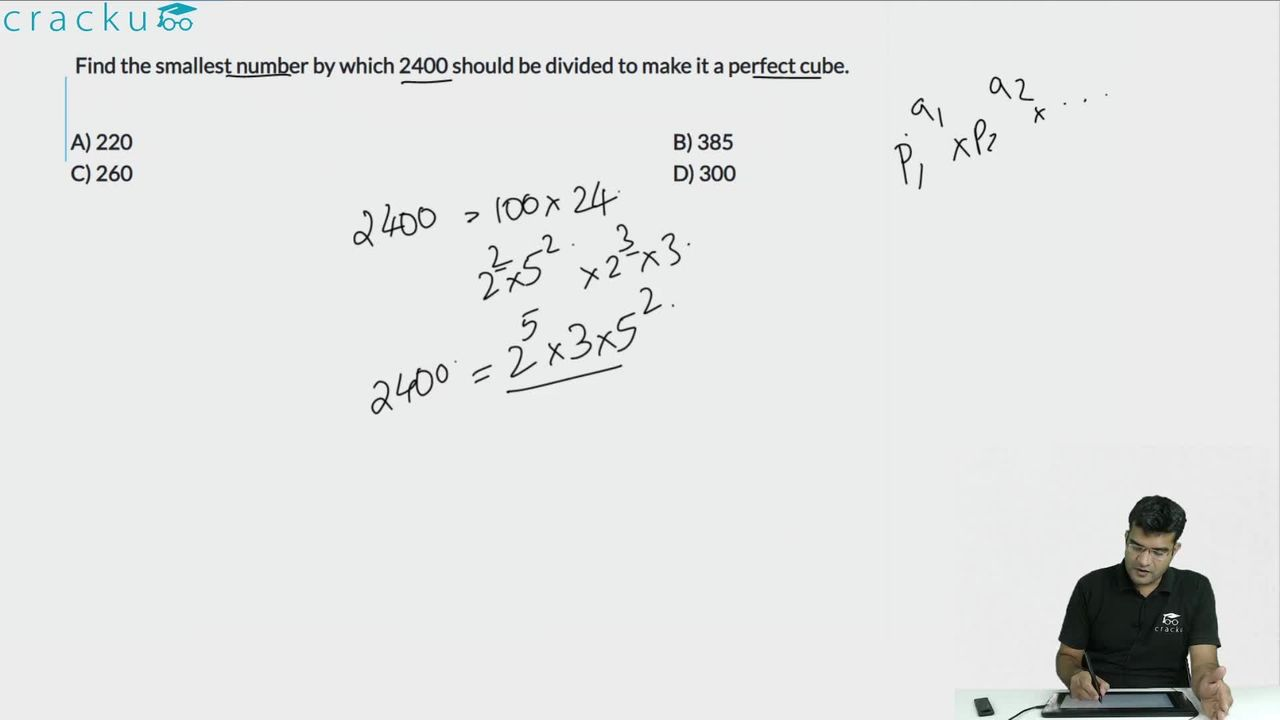Question 3

# Find the smallest number by which 2400 should be divided to make it a perfect cube.

Solution

Prime factorization of :

$$2400=(2)^5\times(3)\times(5)^2$$

Now, to make it a perfect cube, we need to make the powers multiple of 3, and thus we need to divide it by = $$(2)^2\times3\times(5)^2=300$$

=> Ans - (D)

### View Video Solution